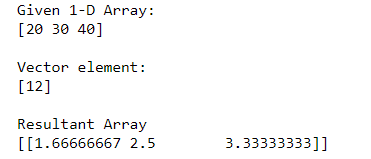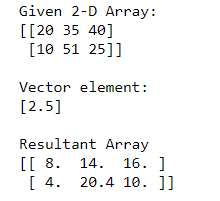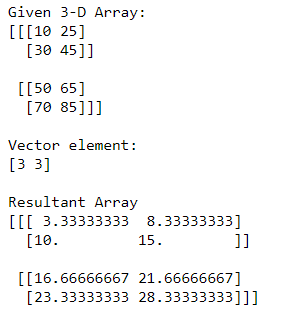# Divide each row by a vector element using NumPy

• Last Updated : 11 Oct, 2020

Prerequisite: Numpy module

The following article depicts how the rows of a Numpy array can be divided by a vector element. The vector element can be a single element, multiple element, or an array. Division operator ( / ) is employed to produce the required functionality.  We can divide rows of 1-D, 2-D, or even more types of arrays with vector element and the following examples will help you understand better:

Attention geek! Strengthen your foundations with the Python Programming Foundation Course and learn the basics.

To begin with, your interview preparations Enhance your Data Structures concepts with the Python DS Course. And to begin with your Machine Learning Journey, join the Machine Learning - Basic Level Course

Example 1:

## Python3

 `# Importing Numpy module ``import` `numpy as np`` ` `# Creating 1-D Numpy array``n_arr ``=` `np.array([``20``, ``30``, ``40``])``print``(``"Given 1-D Array:"``)``print``(n_arr)`` ` `# Vector element ``vec ``=` `np.array([``12``])``print``(``"\nVector element:"``)``print``(vec)`` ` `# Dividing rows of 1-D array with vector element``print``(``"\nResultant Array"``)``print``(n_arr ``/` `vec[:,``None``])`

Output:In the above example, we divide the rows of 1-D Numpy array with vector element i.e 

Example 2:

## Python3

 `# Importing Numpy module ``import` `numpy as np`` ` `# Creating 2-D Numpy array``n_arr ``=` `np.array([[``20``, ``35``, ``40``],``                ``[``10``, ``51``, ``25``]])`` ` `print``(``"Given 2-D Array:"``)``print``(n_arr)`` ` `# Vector element ``vec ``=` `np.array([``2.5``])``print``(``"\nVector element:"``)``print``(vec)`` ` `# Dividing rows of 2-D array with vector element``print``(``"\nResultant Array"``)``print``(n_arr ``/` `vec[:,``None``])`

Output:In the above example, we divide the rows of 2-D Numpy array with vector element i.e [2.5]

Example 3:

## Python3

 `# Importing Numpy module ``import` `numpy as np`` ` `# Creating 3-D Numpy array``n_arr ``=` `np.array([[[``10``, ``25``], [``30``, ``45``]], ``                  ``[[``50``, ``65``], [``70``, ``85``]]])`` ` `print``(``"Given 3-D Array:"``)``print``(n_arr)`` ` `# Vector element ``vec ``=` `np.array([``3``, ``3``])``print``(``"\nVector element:"``)``print``(vec)`` ` `# Dividing rows of 3-D array with vector element``print``(``"\nResultant Array"``)``print``(n_arr ``/` `vec[:,``None``])`

Output:In the above example, we divide the rows of 3-D Numpy array with vector element i.e [3, 3]

My Personal Notes arrow_drop_up